Maths
Study Material

# Linear Differential Equation | Formula | Examples

A linear differential equation is a differential equation, having degree 1, that consists of an independent variable, dependent variable and differential coefficients of dependent variable with respect to independent variable.

7 minutes long
Posted by Kunduz Tutor, 19/10/2021Hesap Oluştur

Got stuck on homework? Get your step-by-step solutions from real tutors in minutes! 24/7. Unlimited.

A differential equation is an equation containing an independent variable, dependent variable and differential coefficients of dependent variable with respect to independent variable, Linear differential equations is a type of differential equation on which we will focus on this blog.

Author – Ojasvi Chaplot

## Introduction to Linear Differential Equation

A linear differential equation is a differential equation, having degree 1, that consists of an independent variable, dependent variable and differential coefficients of dependent variable with respect to independent variable.

Furthermore, a linear equation which consists of one or more terms having the derivatives of the dependent variable with respect to one or more independent variables is called as a linear differential equation(LDE).

## Standard form and Representation of Linear Differential Equation

The standard form and the representation of a LDE is: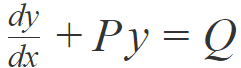This is the linear differential equation formula. Where, P and Q are either numeric constants or functions of x in the LDE.

For Example-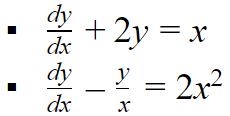## General Terminologies

### Order of a Differential Equation

The order of a differential equation is the order of the highest order derivative appearing in the equation.

For Example –

•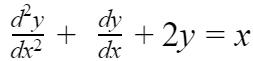; The order of the highest order derivative in the given LDE is 2. So, it is a LDE of order 2.
•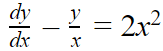; Similarly, in this equation, the order of the highest order derivative is 1. So, it is a LDE of order 1.

GENERAL NOTE: Remember that the order of a LDE (or any differential equation) is a positive integer.

### Degree of Differential Equation

The degree of a differential equation is the degree of the highest order derivative when differential coefficients are made from radicals and fractions.

The degree of a LDE is always 1; otherwise, it is a non-linear differential equation.

For Example –

•In this equation, the degree of the highest order derivative is 1. So, it is a LDE of degree 1.

### Non-Linear Differential Equation

A differential equation is said to be a non-LDE, if:

• The degree of the differential equation is more than 1.
• Any of the differential coefficient has exponent more than 1.
• The exponent of the dependent variable is more than 1.
• The terms containing the products of dependent variable and its differential coefficients are present in the differential equation.

#### Generally, the standard form of a LDE in y is:Where, P and Q are either numeric constants or functions of x in the LDE..

#### And, the standard form of a LDE in x is: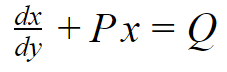Where, P and Q are either numeric constants or functions of y in the LDE.

## Formula for the General Solution of the Linear Differential Equation

The important formulas to find out the general solution of the LDE(s) are listed below.

The general solution of the linear differential equation of the standard formis: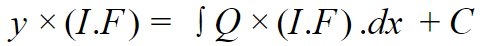Where, C = Arbitrary/Integration Constant

Here, the Integrating Factor,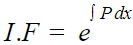The general solution of the linear differential equation of the standard form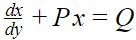is: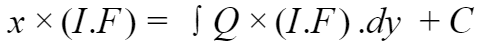Where, C = Arbitrary/Integration Constant

Here, the Integrating Factor,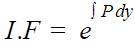## Steps to Find the Solution of a LDE

The steps involved in finding out the solution of the LDE(s) are given as in the following sequence:

• First, rearrange all the terms of the given equation in the standard form of LDE; where P and Q are constants or functions of the independent variable only.
• The step that comes next is to obtain the Integrating Factor (I.F) by using the formula of Integrating Factor (I.F) corresponding to the respective standard form.
• Now, put the value of Integrating Factor (I.F) in the formula for the general solution of a LDE and then solve the obtained equation and simplify it.

Therefore, the solution of the given LDE is obtained.

## Illustrative Examples-

Example-1: Find the solution of the linear differential equation:Solution: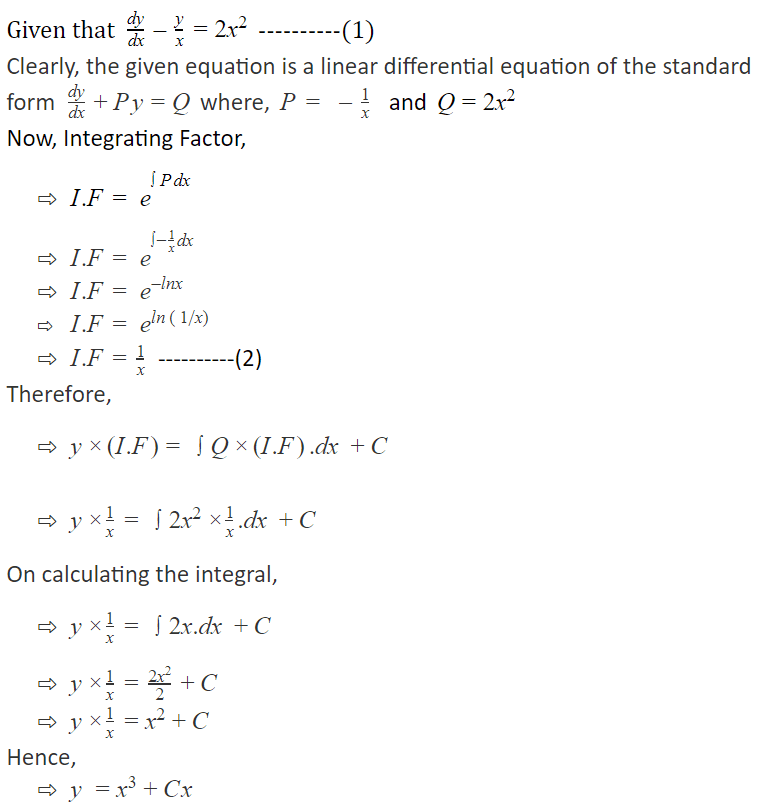Answer:  y =x³+Cx ; where C = Arbitrary/Integration Constant.

Example-2: Solve the LDE :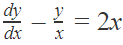Solution:Therefore, Answer:  y =2x²+Cx ; where C = Arbitrary/Integration Constant.

## Picked For You

Furthermore, these are some topics which might interest you: